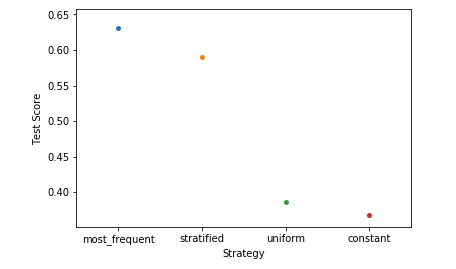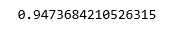# ML | Dummy classifiers using sklearn

A dummy classifier is a type of classifier which does not generate any insight about the data and classifies the given data using only simple rules. The classifier’s behavior is completely independent of the training data as the trends in the training data are completely ignored and instead uses one of the strategies to predict the class label.
It is used only as a simple baseline for the other classifiers i.e. any other classifier is expected to perform better on the given dataset. It is especially useful for datasets where are sure of a class imbalance. It is based on the philosophy that any analytic approach for a classification problem should be better than a random guessing approach.

Below are a few strategies used by the dummy classifier to predict a class label –

1. Most Frequent: The classifier always predicts the most frequent class label in the training data.
2. Stratified: It generates predictions by respecting the class distribution of the training data. It is different from the “most frequent” strategy as it instead associates a probability with each data point of being the most frequent class label.
3. Uniform: It generates predictions uniformly at random.
4. Constant: The classifier always predicts a constant label and is primarily used when classifying non-majority class labels.

Now, let’s see the implementation of dummy classifiers using the sklearn library –

Step 1: Importing the required Libraries

 `import` `numpy as np ` `import` `pandas as pd ` `from` `sklearn.model_selection ``import` `train_test_split ` `from` `sklearn.neighbors ``import` `KNeighborsClassifier ` `import` `matplotlib.pyplot as plt  ` `import` `seaborn as sns `

 `cd C:\Users\Dev\Desktop\Kaggle\Breast_Cancer ` `# Changing the read file location to the location of the file ` `df ``=` `pd.read_csv(``'data.csv'``) ` ` `  `y ``=` `df[``'diagnosis'``] ` `X ``=` `df.drop(``'diagnosis'``, axis ``=` `1``) ` `X ``=` `X.drop(``'Unnamed: 32'``, axis ``=` `1``) ` `X ``=` `X.drop(``'id'``, axis ``=` `1``) ` `# Separating the dependent and independent variable ` ` `  `X_train, X_test, y_train, y_test ``=` `train_test_split( ` `            ``X, y, test_size ``=` `0.3``, random_state ``=` `0``) ` `# Splitting the data into training and testing data `

Step 3: Training the dummy model

 `strategies ``=` `[``'most_frequent'``, ``'stratified'``, ``'uniform'``, ``'constant'``] ` ` `  `test_scores ``=` `[] ` `for` `s ``in` `strategies: ` `    ``if` `s ``=``=``'constant'``: ` `        ``dclf ``=` `DummyClassifier(strategy ``=` `s, random_state ``=` `0``, constant ``=``'M'``) ` `    ``else``: ` `        ``dclf ``=` `DummyClassifier(strategy ``=` `s, random_state ``=` `0``) ` `    ``dclf.fit(X_train, y_train) ` `    ``score ``=` `dclf.score(X_test, y_test) ` `    ``test_scores.append(score) `

Step 4: Analyzing our results

 `ax ``=` `sns.stripplot(strategies, test_scores); ` `ax.``set``(xlabel ``=``'Strategy'``, ylabel ``=``'Test Score'``) ` `plt.show() `Step 5: Training the KNN model

 `clf ``=` `KNeighborsClassifier(n_neighbors ``=` `5``) ` `clf.fit(X_train, y_train) ` `print``(clf.score(X_test, y_test)) `On comparing the scores of the KNN classifier with the dummy classifier, we come to the conclusion that the KNN classifier is, in fact, a good classifier for the given data.

My Personal Notes arrow_drop_upCheck out this Author's contributed articles.

If you like GeeksforGeeks and would like to contribute, you can also write an article using contribute.geeksforgeeks.org or mail your article to contribute@geeksforgeeks.org. See your article appearing on the GeeksforGeeks main page and help other Geeks.

Please Improve this article if you find anything incorrect by clicking on the "Improve Article" button below.

Improved By : AlindGupta, shubham_singh

Article Tags :
Practice Tags :

1

Please write to us at contribute@geeksforgeeks.org to report any issue with the above content.